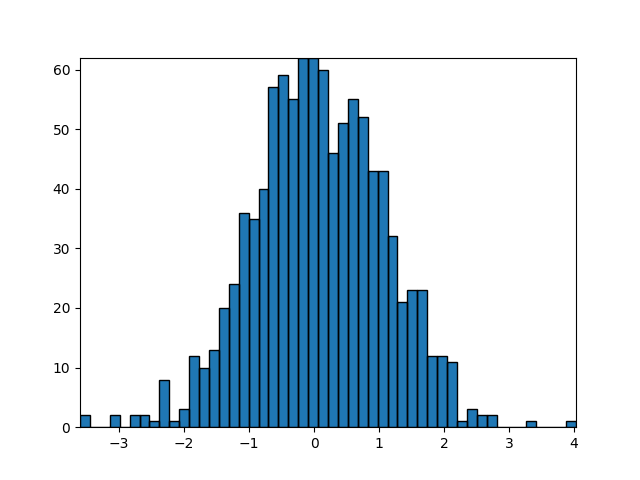Building histograms using Rectangles and PolyCollections¶

Using a path patch to draw rectangles. The technique of using lots of Rectangle instances, or the faster method of using PolyCollections, were implemented before we had proper paths with moveto/lineto, closepoly etc in mpl. Now that we have them, we can draw collections of regularly shaped objects with homogeneous properties more efficiently with a PathCollection. This example makes a histogram -- it's more work to set up the vertex arrays at the outset, but it should be much faster for large numbers of objects.

import numpy as np
import matplotlib.pyplot as plt
import matplotlib.patches as patches
import matplotlib.path as path

fig, ax = plt.subplots()

# Fixing random state for reproducibility
np.random.seed(19680801)

# histogram our data with numpy

data = np.random.randn(1000)
n, bins = np.histogram(data, 50)

# get the corners of the rectangles for the histogram
left = bins[:-1]
right = bins[1:]
bottom = np.zeros(len(left))
top = bottom + n

# we need a (numrects x numsides x 2) numpy array for the path helper
# function to build a compound path
XY = np.array([[left, left, right, right], [bottom, top, top, bottom]]).T

# get the Path object
barpath = path.Path.make_compound_path_from_polys(XY)

# make a patch out of it
patch = patches.PathPatch(barpath)

# update the view limits
ax.set_xlim(left, right[-1])
ax.set_ylim(bottom.min(), top.max())

plt.show()It should be noted that instead of creating a three-dimensional array and using make_compound_path_from_polys, we could as well create the compound path directly using vertices and codes as shown below

nrects = len(left)
nverts = nrects*(1+3+1)
verts = np.zeros((nverts, 2))
codes = np.ones(nverts, int) * path.Path.LINETO
codes[0::5] = path.Path.MOVETO
codes[4::5] = path.Path.CLOSEPOLY
verts[0::5, 0] = left
verts[0::5, 1] = bottom
verts[1::5, 0] = left
verts[1::5, 1] = top
verts[2::5, 0] = right
verts[2::5, 1] = top
verts[3::5, 0] = right
verts[3::5, 1] = bottom

barpath = path.Path(verts, codes)